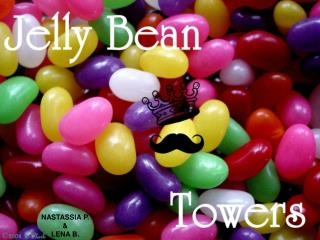# NASTASSIA P. & LENA B. - PowerPoint PPT PresentationDownload PresentationNASTASSIA P. & LENA B.

NASTASSIA P. & LENA B.Download Presentation## NASTASSIA P. & LENA B.

- - - - - - - - - - - - - - - - - - - - - - - - - - - E N D - - - - - - - - - - - - - - - - - - - - - - - - - - -
##### Presentation Transcript

1. NASTASSIA P. & LENA B.

2. THE QUESTION • How many buildings the size of the CN Tower would a mole of jelly beans fill up?

3. THE GIVEN • A mole = 6.0221415 × 10^23 mol^-1 • Average volume of jelly bean: 0.015m x 0.01m x 0.01m = 0.0000015m^3 • Volume of Cone: 1/3 π r^2 h • Radius at the base of the tower = 33.2m • Height of the CN Tower = 553.33m • Main level floor is 256 square feet • Main level height = 7 stories • 1 story = 10 feet • 1 foot = 0.3048 meters • Main level height = 7 x 10 x 0.3048 = 21.336m Height of 553.55m }7 stories } Radius of 33.2m

4. THE CALCULATIONS Find volume of the “cone” part. Name it Volume C. • Volume of Cone: 1/3 π (33.2m)^2 x (553.33m) • Volume C = around 638 688m^3 Find volume of Sky Pod. Name it Volume P. • Volume of Sky Pod = 21.336m x 256m^2 • Volume P = around 5462m^3 Total volume of CN Tower = Volume C + Volume P • Total volume = 638 688 + 5462 • Total volume = 644 150m^3

5. THE CALCULATIONS Multiply Avagadro’s consant by volume of jelly bean. Divide it by volume of CN Tower. • # of CN Towers = Avogadro’s constant x volume of jelly bean volume of 1 CN Tower • Number of CN Towers = (6.022 x 10^23)(0.0000015) 644 150 • Number of CN Towers = 1.402313126 x 10^12 = 1 402 313 126 000

6. THE END Therefore, 1 402 313 126 000 buildings the size of the CN Tower will fill up a mole of jelly beans.